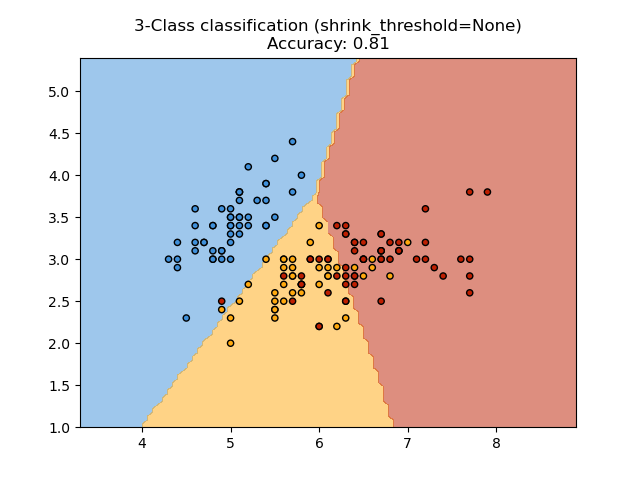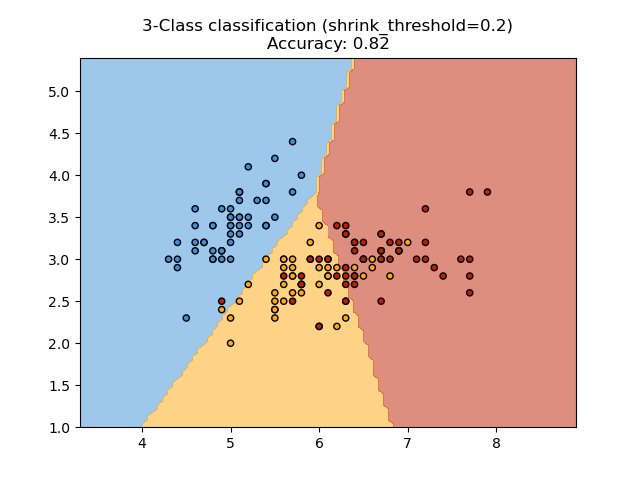# Nearest Centroid Classification¶

Sample usage of Nearest Centroid classification. It will plot the decision boundaries for each class.

••None 0.8133333333333334
0.2 0.82


import numpy as np
import matplotlib.pyplot as plt
from matplotlib.colors import ListedColormap
from sklearn import datasets
from sklearn.neighbors import NearestCentroid
from sklearn.inspection import DecisionBoundaryDisplay

n_neighbors = 15

# import some data to play with
# we only take the first two features. We could avoid this ugly
# slicing by using a two-dim dataset
X = iris.data[:, :2]
y = iris.target

# Create color maps
cmap_light = ListedColormap(["orange", "cyan", "cornflowerblue"])
cmap_bold = ListedColormap(["darkorange", "c", "darkblue"])

for shrinkage in [None, 0.2]:
# we create an instance of Neighbours Classifier and fit the data.
clf = NearestCentroid(shrink_threshold=shrinkage)
clf.fit(X, y)
y_pred = clf.predict(X)
print(shrinkage, np.mean(y == y_pred))

_, ax = plt.subplots()
DecisionBoundaryDisplay.from_estimator(
clf, X, cmap=cmap_light, ax=ax, response_method="predict"
)

# Plot also the training points
plt.scatter(X[:, 0], X[:, 1], c=y, cmap=cmap_bold, edgecolor="k", s=20)
plt.title("3-Class classification (shrink_threshold=%r)" % shrinkage)
plt.axis("tight")

plt.show()


Total running time of the script: ( 0 minutes 0.196 seconds)

Gallery generated by Sphinx-Gallery